# How to acess direction information of drone using flight records

Hello,

How can I access drone direction information when it goes. Maybe there is a compass information. However, I did not see any information in flight records. Or is it possible to calculate, find its direction using other data. Maybe prior coordinates?

Best regards.

The “OSD.yaw ” value shows the aircraft direction in degrees.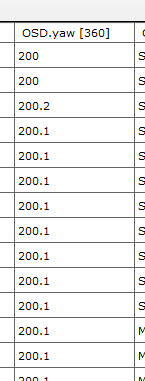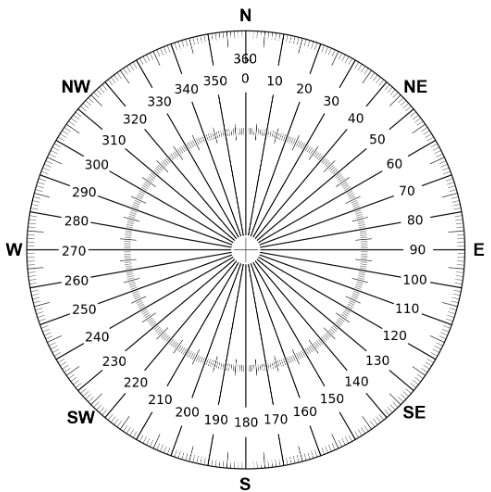1 Like

@msinger So much tanks for this information.

Best regards.

@msinger how can I transform these degree to unit latitude and longtitude direction. For example, if yaw degree is 270 then your coordinate will be changed from 38.10, 43.27 to 38.05, 43.27

I believe that you’re referring to direction of travel and not heading. The drone can and usually does fly at least partially sideways. Here is the code that DatCon uses to compute direction of travel

// computes true(not magnetic) bearing
public static double bearing(double lat1, double lon1, double lat2,
double lon2) {
double longDiff = (lon2 - lon1);
double y = Math.sin(longDiff) * Math.cos(lat2);
double x = Math.cos(lat1) * Math.sin(lat2)
- Math.sin(lat1) * Math.cos(lat2) * Math.cos(longDiff);
return Math.toDegrees(Math.atan2(y, x));
}

@BudWalker I think I need to heading direction. My drone is going or it stays at somewhere. I would like to know where drone or gimbal is looking at. Then I can gcalculate a new coordinate using this point of view and a distance. And this new coordinate belongs to distance point.

Assume I have a distance and it is 450 and my looking direction is 310 degree. Then it means my new coordinate would maybe (f(310, 450)+ latitude), (f(310,450) + lontitude). What should this f function be?

I made some research and find this post. I am not sure of whether it is right or not. However, when I use, it works some cases but I also think does not work for some cases.
Here a simple example:

yaw_angle = 310
distance = 450

lan = 37.4815207267064
lon = 38.6497129186169

lan_direction = -sin(yaw_angle)
lon_direction = -cos(yaw_angle)

dx = (lan_direction* distance)
dy = (lon_direction* distance)

r_earth = 6378000 #unit is meter and means radius of earth
object_lan = lan + (dy / r_earth) * (180 / pi)
object_lon = lon + (dx / r_earth) * (180 / pi) / cos(lan * pi/180)

if I calculate all of them, object_lan should be 37.48364444748233 and object_lon should be 38.6453783211518

(f(310, 450)+ latitude), (f(310,450) + lontitude) can’t be correct since the function f has to be dependent on latitude and longitude

Try the following code
* Math.cos(brng));

I haven’t tested it but I think it’s right. For your example
brng = 310
distance = 450

and the answer I get is
long = 38.65405215515889
lat = 37.47939459319298

So much thanks for your all helps. I made some calculations. However, I see its direction is not right.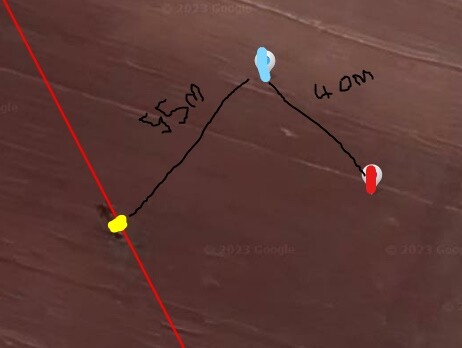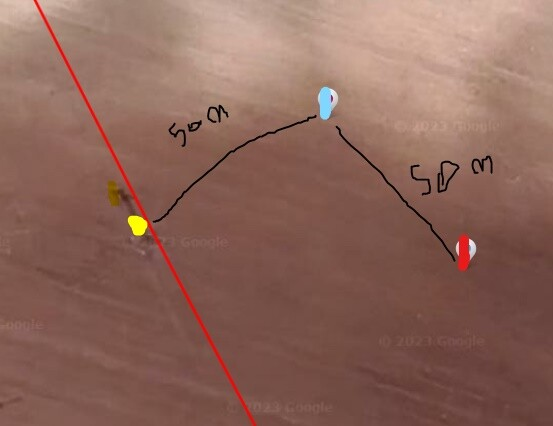Look at two picture. Blue one is position of drone, red one calculated position and yellow one actual position. It seems there is 90 degree offset.

Best regards.

I believe the problem is that brng should be in radians not degrees. To achieve that multiply degrees by .01745, i.e.

``````    double destLongRadians = longRadians + Math.atan2(

``````

@BudWalker I tried to convert it to radian. However, unfortunatelly there is problem again. I added two images.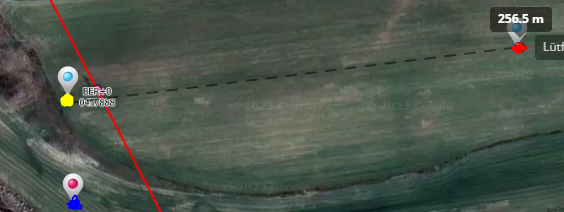blue one indicate my calculation, red one your calculation and yellow one actual position. Again there is a shifting.

Best regards.

I suspect that your code has a bug. Here is my Java code

``````public static void destnation(double latitudeDegrees,
double longitudeDegrees, double brng, double distance) {

System.out.println("Origin Lat = " + latitudeDegrees + " Long = "
+ longitudeDegrees + " brng = " + brng + " distance = "
+ distance);

System.out.println("Destination Lat = " + destLatDegrees + " Long = "
+ destLongDegrees);
}

public static void main(String[] args) {
Util.destnation(37.4815207267064, 38.6497129186169, 310, 450);
}
``````

and it’s output

Origin Lat = 37.4815207267064 Long = 38.6497129186169 brng = 310.0 distance = 450.0
Destination Lat = 37.48412198992392 Long = 38.64580610647096

You can compile and execute this code. Or, you could provide your inputs and I’ll run the code

Thank you for your response. Yes, you are right. I realized, my codes convert degrees to radians twice. I have some questions but I will create a new topic about them.

Thank you for all your helps.
Best regards.